# Chapter 5: Prediction

## Section 4.1: Predicting Election Outcomes

### Section 4.1.1: Loops in R

values <- c(2, 4, 6)
n <- length(values) # number of elements in values'
results <- rep(NA, n) # empty container vector for storing the results

## loop counter i' will take values on 1, 2, ..., n in that order
for (i in 1:n) {
## store the result of multiplication as the ith element of
## results' vector
results[i] <- values[i] * 2
cat(values[i], "times 2 is equal to", results[i], "\n")
}
## 2 times 2 is equal to 4
## 4 times 2 is equal to 8
## 6 times 2 is equal to 12
results
##   4  8 12
## check if the code runs when i = 1
i <- 1
x <- values[i] * 2
cat(values[i], "times 2 is equal to", x, "\n")
## 2 times 2 is equal to 4

### Section 4.1.2: General Conditional Statements in R

## define the operation to be executed
cat("I will perform addition 4 + 4\n")
4 + 4
}
## I will perform addition 4 + 4
##  8
if (operation == "multiply") {
cat("I will perform multiplication 4 * 4\n")
4 * 4
}

## Note that operation' is redefined
operation <- "multiply"
cat("I will perform addition 4 + 4")
4 + 4
} else {
cat("I will perform multiplication 4 * 4")
4 * 4
}
## I will perform multiplication 4 * 4
##  16
## Note that operation' is redefined
operation <- "subtract"
cat("I will perform addition 4 + 4\n")
4 + 4
} else if (operation == "multiply") {
cat("I will perform multiplication 4 * 4\n")
4 * 4
} else {
cat("", operation, "' is invalid. Use either add' or multiply'.\n",
sep = "")
}
## subtract' is invalid. Use either add' or multiply'.
values <- 1:5
n <-  length(values)
results <- rep(NA, n)
for (i in 1:n) {
## x and r get overwritten in each iteration
x <- values[i]
r <- x %% 2  # remainder when divided by 2 to check whether even or odd
if (r == 0) { # remainder is zero
cat(x, "is even and I will perform addition",
x, "+", x, "\n")
results[i] <- x + x
} else { # remainder is not zero
cat(x, "is odd and I will perform multiplication",
x, "*", x, "\n")
results[i] <- x * x
}
}
## 1 is odd and I will perform multiplication 1 * 1
## 2 is even and I will perform addition 2 + 2
## 3 is odd and I will perform multiplication 3 * 3
## 4 is even and I will perform addition 4 + 4
## 5 is odd and I will perform multiplication 5 * 5
results
##   1  4  9  8 25

### Section 4.1.3: Poll Predictions

## load election results, by state
data("pres08", package = "qss")
data("polls08", package = "qss")
## compute Obama's margin
polls08$margin <- polls08$Obama - polls08$McCain pres08$margin <- pres08$Obama - pres08$McCain

x <- as.Date("2008-11-04")
## Warning in strptime(xx, f <- "%Y-%m-%d", tz = "GMT"): unknown timezone
## 'zone/tz/2017c.1.0/zoneinfo/America/New_York'
y <- as.Date("2008/9/1")
x - y # number of days between 2008/9/1 and 11/4
## Time difference of 64 days
## convert to a Date object
polls08$middate <- as.Date(polls08$middate)

## computer the number of days to the election day
polls08$DaysToElection <- as.Date("2008-11-04") - polls08$middate
poll.pred <- rep(NA, 51) # initialize a vector place holder

## extract unique state names which the loop will iterate through
st.names <- unique(polls08$state) ## add state names as labels for easy interpretation later on names(poll.pred) <- as.character(st.names) ## loop across 50 states plus DC for (i in 1:51){ ## subset the ith state state.data <- subset(polls08, subset = (state == st.names[i])) ## further subset the latest polls within the state latest <- subset(state.data, DaysToElection == min(DaysToElection)) ## compute the mean of latest polls and store it poll.pred[i] <- mean(latest$margin)
}

## error of latest polls
errors <- pres08$margin - poll.pred names(errors) <- st.names # add state names mean(errors) # mean prediction error ##  1.062092 sqrt(mean(errors^2)) ##  5.90894 par(cex = 1.5) ## histogram hist(errors, freq = FALSE, ylim = c(0, 0.08), main = "Poll prediction error", xlab = "Error in predicted margin for Obama (percentage points)") ## add mean abline(v = mean(errors), lty = "dashed", col = "red") text(x = -7, y = 0.07, "average error", col = "red")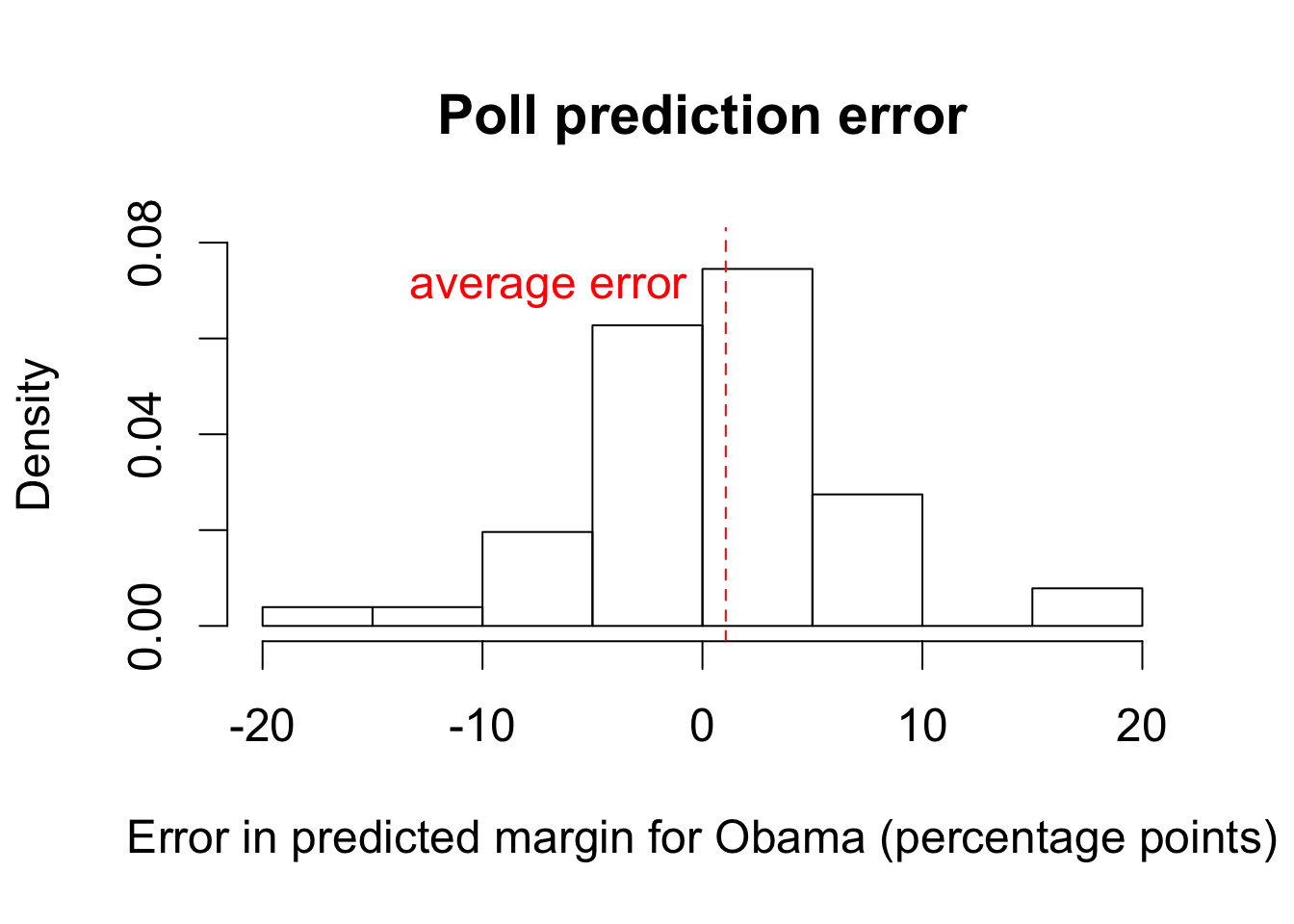par(cex = 1.5) ## type = "n" generates "empty" plot plot(poll.pred, pres08$margin, type = "n", main = "", xlab = "Poll results",
xlim = c(-40, 90), ylim = c(-40, 90), ylab = "Actual election results")
text(x = poll.pred, y = pres08$margin, labels = pres08$state, col = "blue")
## lines
abline(a = 0, b = 1, lty = "dashed") # 45 degree line
abline(v = 0)  # vertical line at 0
abline(h = 0)  # horizontal line at 0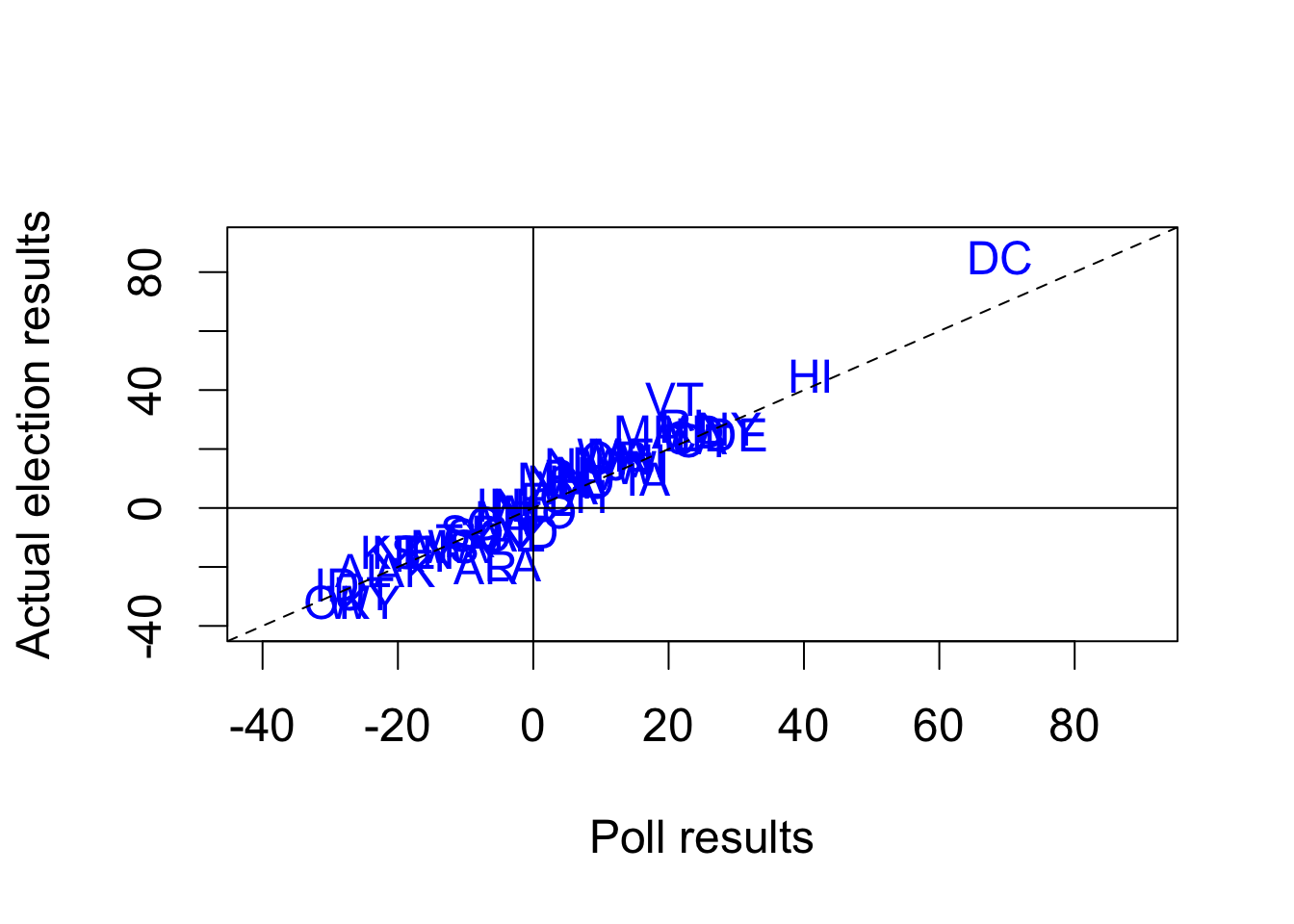## which state polls called wrong?
pres08$state[sign(poll.pred) != sign(pres08$margin)]
##  "IN" "MO" "NC"
## what was the actual margin for these states?
pres08$margin[sign(poll.pred) != sign(pres08$margin)]
##   1 -1  1
## actual results: total number of electoral votes won by Obama
sum(pres08$EV[pres08$margin > 0])
##  364
## poll prediction
sum(pres08$EV[poll.pred > 0]) ##  349 ## load the data data("pollsUS08", package = "qss") ## compute number of days to the election as before pollsUS08$middate <- as.Date(pollsUS08$middate) pollsUS08$DaysToElection <- as.Date("2008-11-04") - pollsUS08$middate ## empty vectors to store predictions Obama.pred <- McCain.pred <- rep(NA, 90) for (i in 1:90) { ## take all polls conducted within the past 7 days week.data <- subset(pollsUS08, subset = ((DaysToElection <= (90 - i + 7)) & (DaysToElection > (90 - i)))) ## compute support for each candidate using the average Obama.pred[i] <- mean(week.data$Obama)
McCain.pred[i] <- mean(week.data$McCain) } par(cex = 1.5) ## plot going from 90 days to 1 day before the election plot(90:1, Obama.pred, type = "b", xlim = c(90, 0), ylim = c(40, 60), col = "blue", xlab = "Days to the election", ylab = "Support for candidate (percentage points)") ## type = "b"' gives plot that includes both points and lines lines(90:1, McCain.pred, type = "b", col = "red") ## actual election results: pch = 19 gives solid circles points(0, 52.93, pch = 19, col = "blue") points(0, 45.65, pch = 19, col = "red") ## line indicating the election day abline(v = 0) ## labeling candidates text(80, 48, "Obama", col = "blue") text(80, 41, "McCain", col = "red")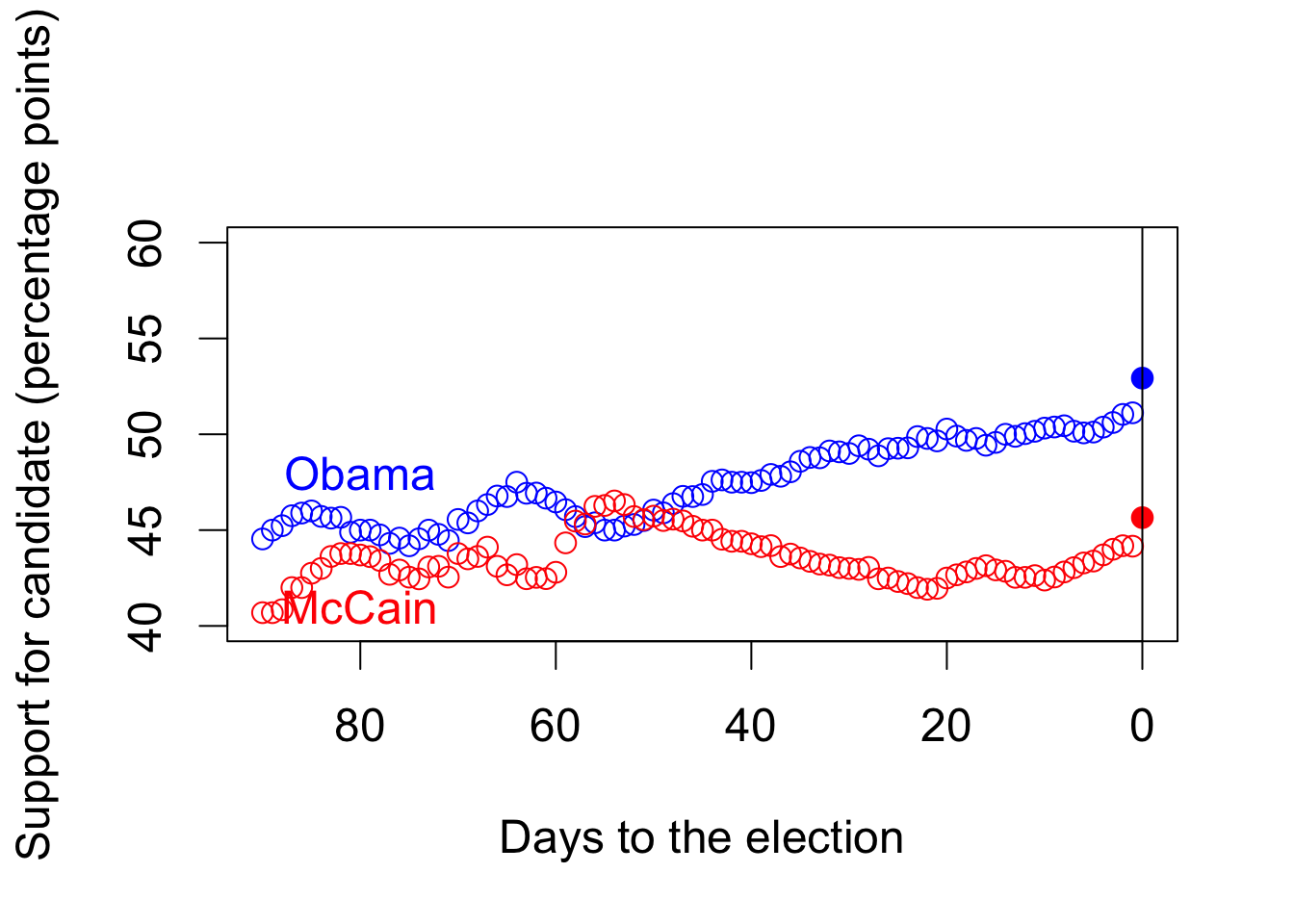## Section 4.2: Linear Regression ### Section 4.2.1: Facial Appearance and Election Outcomes ## load the data data("face", package = "qss") ## two-party vote share for Democrats and Republicans face$d.share <- face$d.votes / (face$d.votes + face$r.votes) face$r.share <- face$r.votes / (face$d.votes + face$r.votes) face$diff.share <- face$d.share - face$r.share

par(cex = 1.5)
plot(face$d.comp, face$diff.share, pch = 16,
col = ifelse(face$w.party == "R", "red", "blue"), xlim = c(0, 1), ylim = c(-1, 1), xlab = "Competence scores for Democrats", ylab = "Democratic margin in vote share", main = "Facial competence and vote share")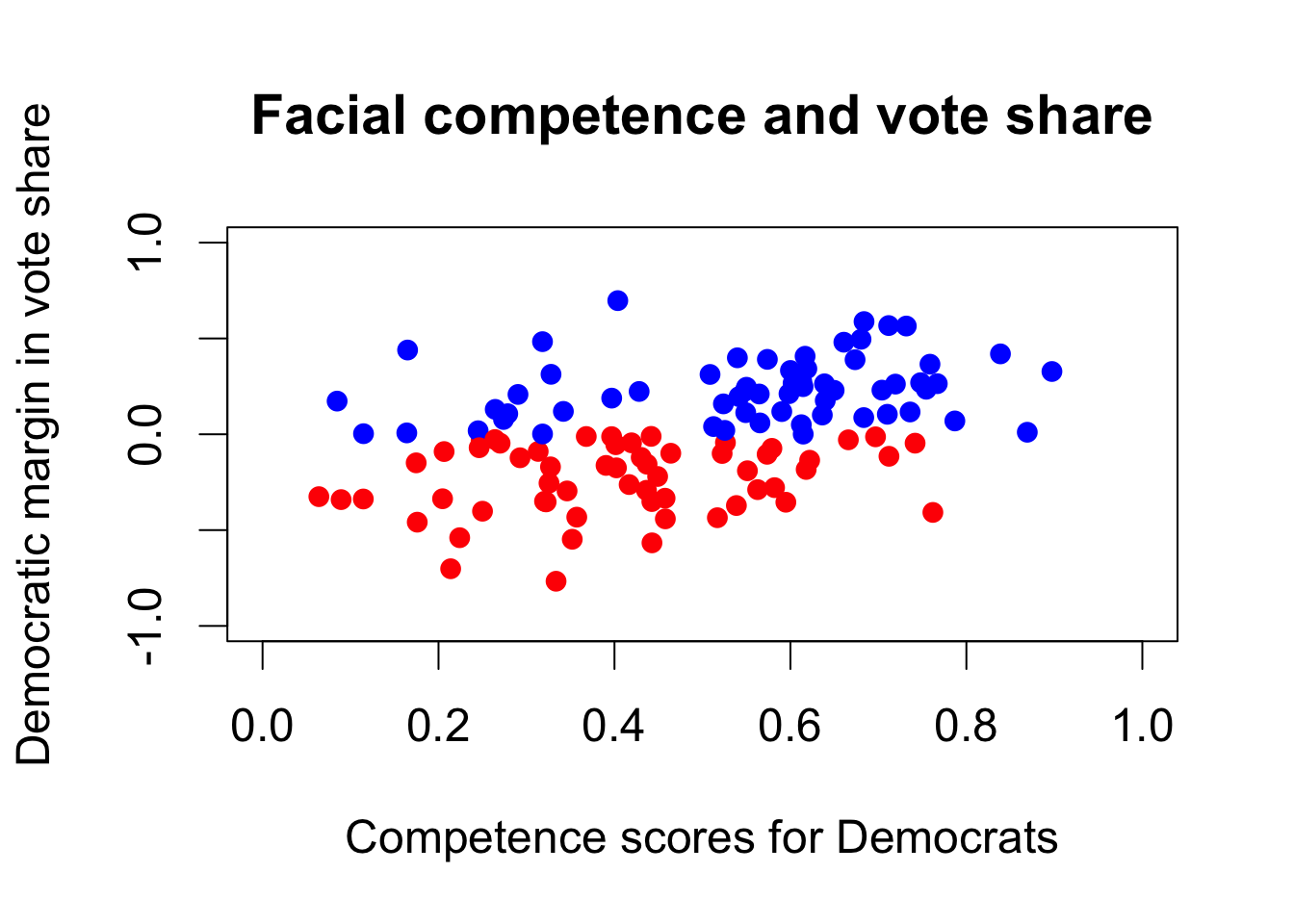### Section 4.2.2: Correlation and Scatter Plots cor(face$d.comp, face$diff.share) ##  0.4327743 ### Section 4.2.3: Least Squares fit <- lm(diff.share ~ d.comp, data = face) # fit the model fit ## ## Call: ## lm(formula = diff.share ~ d.comp, data = face) ## ## Coefficients: ## (Intercept) d.comp ## -0.3122 0.6604 ## lm(face$diff.share ~ face$d.comp) coef(fit) # get estimated coefficients ## (Intercept) d.comp ## -0.3122259 0.6603815 head(fitted(fit)) # get fitted or predicted values ## 1 2 3 4 5 6 ## 0.06060411 -0.08643340 0.09217061 0.04539236 0.13698690 -0.10057206 plot(face$d.comp, face$diff.share, xlim = c(0, 1.05), ylim = c(-1,1), xlab = "Competence scores for Democrats", ylab = "Democratic margin in vote share", main = "Facial competence and vote share") abline(fit) # add regression line abline(v = 0, lty = "dashed")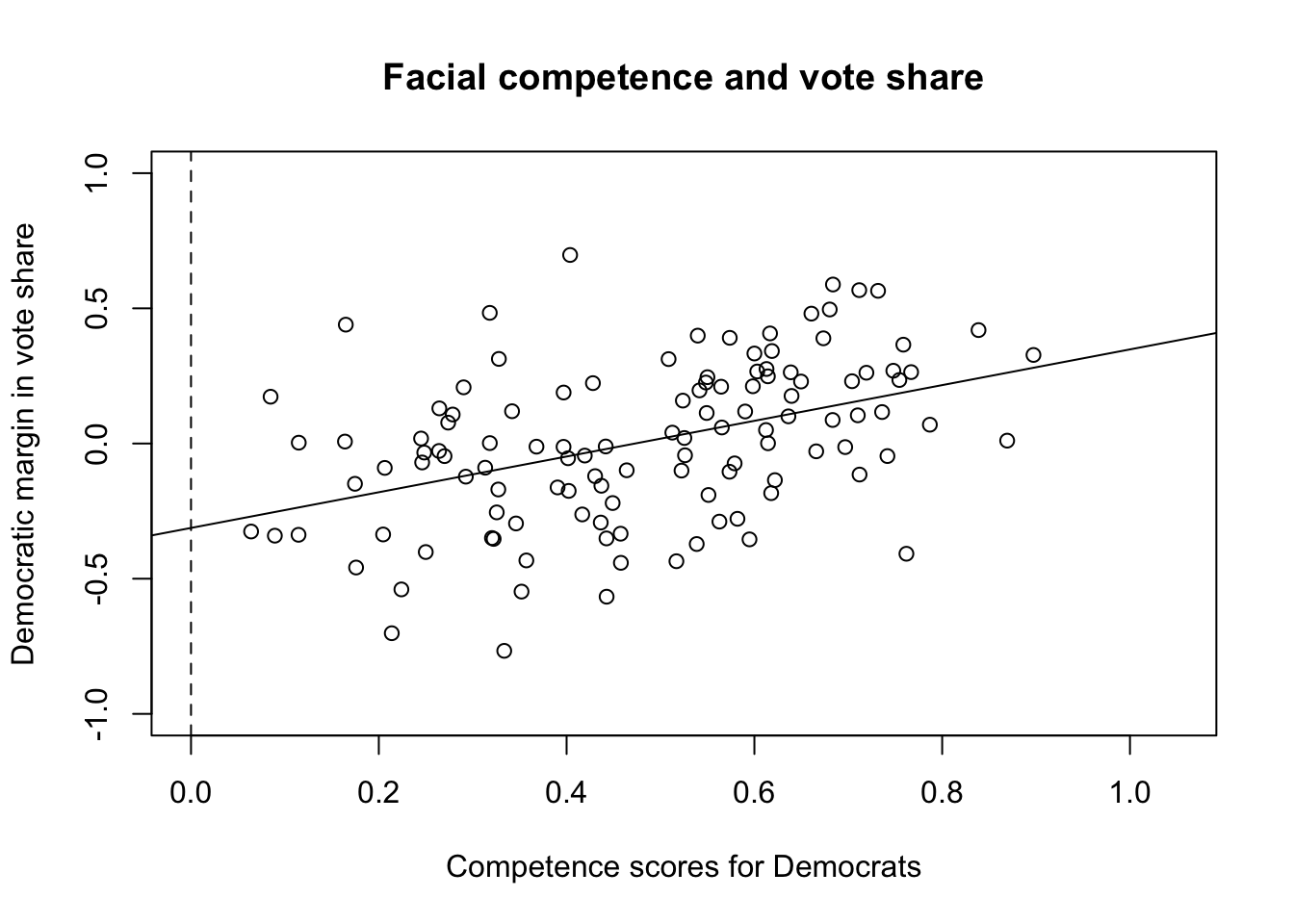epsilon.hat <- resid(fit) # residuals sqrt(mean(epsilon.hat^2)) # RMSE ##  0.2642361 ### Section 4.2.4: Regression Towards the Mean ### Section 4.2.5: Merging Data Sets in R data("pres12", package = "qss") # load 2012 data ## quick look at two data sets head(pres08) ## state.name state Obama McCain EV margin ## 1 Alabama AL 39 60 9 -21 ## 2 Alaska AK 38 59 3 -21 ## 3 Arizona AZ 45 54 10 -9 ## 4 Arkansas AR 39 59 6 -20 ## 5 California CA 61 37 55 24 ## 6 Colorado CO 54 45 9 9 head(pres12) ## state Obama Romney EV ## 1 AL 38 61 9 ## 2 AK 41 55 3 ## 3 AZ 45 54 11 ## 4 AR 37 61 6 ## 5 CA 60 37 55 ## 6 CO 51 46 9 ## merge two data frames pres <- merge(pres08, pres12, by = "state") ## summarize the merged data frame summary(pres) ## state state.name Obama.x McCain ## Length:51 Length:51 Min. :33.00 Min. : 7.00 ## Class :character Class :character 1st Qu.:43.00 1st Qu.:40.00 ## Mode :character Mode :character Median :51.00 Median :47.00 ## Mean :51.37 Mean :47.06 ## 3rd Qu.:57.50 3rd Qu.:56.00 ## Max. :92.00 Max. :66.00 ## EV.x margin Obama.y Romney ## Min. : 3.00 Min. :-32.000 Min. :25.00 Min. : 7.00 ## 1st Qu.: 4.50 1st Qu.:-13.000 1st Qu.:40.50 1st Qu.:41.00 ## Median : 8.00 Median : 4.000 Median :51.00 Median :48.00 ## Mean :10.55 Mean : 4.314 Mean :49.06 Mean :49.04 ## 3rd Qu.:11.50 3rd Qu.: 17.500 3rd Qu.:56.00 3rd Qu.:58.00 ## Max. :55.00 Max. : 85.000 Max. :91.00 Max. :73.00 ## EV.y ## Min. : 3.00 ## 1st Qu.: 4.50 ## Median : 8.00 ## Mean :10.55 ## 3rd Qu.:11.50 ## Max. :55.00 ## change the variable name for illustration names(pres12) <- "state.abb" ## merging data sets using the variables of different names pres <- merge(pres08, pres12, by.x = "state", by.y = "state.abb") summary(pres) ## state state.name Obama.x McCain ## Length:51 Length:51 Min. :33.00 Min. : 7.00 ## Class :character Class :character 1st Qu.:43.00 1st Qu.:40.00 ## Mode :character Mode :character Median :51.00 Median :47.00 ## Mean :51.37 Mean :47.06 ## 3rd Qu.:57.50 3rd Qu.:56.00 ## Max. :92.00 Max. :66.00 ## EV.x margin Obama.y Romney ## Min. : 3.00 Min. :-32.000 Min. :25.00 Min. : 7.00 ## 1st Qu.: 4.50 1st Qu.:-13.000 1st Qu.:40.50 1st Qu.:41.00 ## Median : 8.00 Median : 4.000 Median :51.00 Median :48.00 ## Mean :10.55 Mean : 4.314 Mean :49.06 Mean :49.04 ## 3rd Qu.:11.50 3rd Qu.: 17.500 3rd Qu.:56.00 3rd Qu.:58.00 ## Max. :55.00 Max. : 85.000 Max. :91.00 Max. :73.00 ## EV.y ## Min. : 3.00 ## 1st Qu.: 4.50 ## Median : 8.00 ## Mean :10.55 ## 3rd Qu.:11.50 ## Max. :55.00 ## cbinding two data frames pres1 <- cbind(pres08, pres12) ## this shows all variables are kept summary(pres1) ## state.name state Obama McCain ## Length:51 Length:51 Min. :33.00 Min. : 7.00 ## Class :character Class :character 1st Qu.:43.00 1st Qu.:40.00 ## Mode :character Mode :character Median :51.00 Median :47.00 ## Mean :51.37 Mean :47.06 ## 3rd Qu.:57.50 3rd Qu.:56.00 ## Max. :92.00 Max. :66.00 ## EV margin state.abb Obama ## Min. : 3.00 Min. :-32.000 Length:51 Min. :25.00 ## 1st Qu.: 4.50 1st Qu.:-13.000 Class :character 1st Qu.:40.50 ## Median : 8.00 Median : 4.000 Mode :character Median :51.00 ## Mean :10.55 Mean : 4.314 Mean :49.06 ## 3rd Qu.:11.50 3rd Qu.: 17.500 3rd Qu.:56.00 ## Max. :55.00 Max. : 85.000 Max. :91.00 ## Romney EV ## Min. : 7.00 Min. : 3.00 ## 1st Qu.:41.00 1st Qu.: 4.50 ## Median :48.00 Median : 8.00 ## Mean :49.04 Mean :10.55 ## 3rd Qu.:58.00 3rd Qu.:11.50 ## Max. :73.00 Max. :55.00 ## DC and DE are flipped in this alternative approach pres1[8:9, ] ## state.name state Obama McCain EV margin state.abb Obama Romney EV ## 8 D.C. DC 92 7 3 85 DE 59 40 3 ## 9 Delaware DE 62 37 3 25 DC 91 7 3 ## merge() does not have this problem pres[8:9, ] ## state state.name Obama.x McCain EV.x margin Obama.y Romney EV.y ## 8 DC D.C. 92 7 3 85 91 7 3 ## 9 DE Delaware 62 37 3 25 59 40 3 pres$Obama2008.z <- scale(pres$Obama.x) pres$Obama2012.z <- scale(pres$Obama.y) ## intercept is estimated essentially zero fit1 <- lm(Obama2012.z ~ Obama2008.z, data = pres) fit1 ## ## Call: ## lm(formula = Obama2012.z ~ Obama2008.z, data = pres) ## ## Coefficients: ## (Intercept) Obama2008.z ## -3.521e-17 9.834e-01 ## regression without an intercept; estimated slope is identical fit1 <- lm(Obama2012.z ~ -1 + Obama2008.z, data = pres) fit1 ## ## Call: ## lm(formula = Obama2012.z ~ -1 + Obama2008.z, data = pres) ## ## Coefficients: ## Obama2008.z ## 0.9834 par(cex = 1.5) plot(pres$Obama2008.z, pres$Obama2012.z, xlim = c(-4, 4), ylim = c(-4, 4), xlab = "Obama's standardized vote share in 2008", ylab = "Obama's standardized vote share in 2012") abline(fit1) # draw a regression line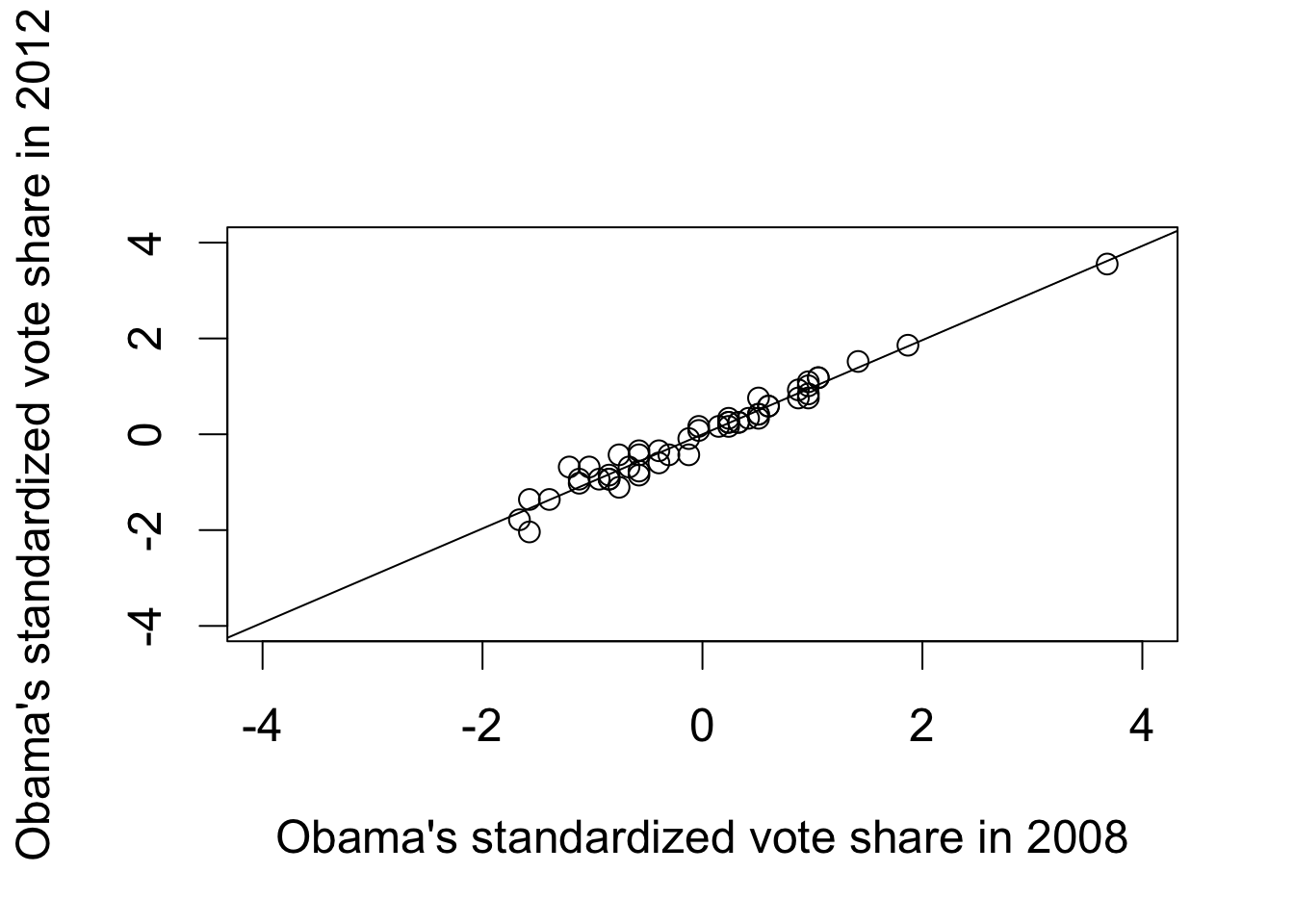## bottom quartile mean((pres$Obama2012.z >
pres$Obama2008.z)[pres$Obama2008.z
<= quantile(pres$Obama2008.z, 0.25)]) ##  0.5714286 ## top quartile mean((pres$Obama2012.z >
pres$Obama2008.z)[pres$Obama2008.z
>= quantile(pres$Obama2008.z, 0.75)]) ##  0.4615385 ### Section 4.2.6: Model Fit data("florida", package = "qss") ## regress Buchanan's 2000 votes on Perot's 1996 votes fit2 <- lm(Buchanan00 ~ Perot96, data = florida) fit2 ## ## Call: ## lm(formula = Buchanan00 ~ Perot96, data = florida) ## ## Coefficients: ## (Intercept) Perot96 ## 1.34575 0.03592 ## compute TSS (total sum of squares) and SSR (sum of squared residuals) TSS2 <- sum((florida$Buchanan00 - mean(florida$Buchanan00))^2) SSR2 <- sum(resid(fit2)^2) ## Coefficient of determination (TSS2 - SSR2) / TSS2 ##  0.5130333 R2 <- function(fit) { resid <- resid(fit) # residuals y <- fitted(fit) + resid # outcome variable TSS <- sum((y - mean(y))^2) SSR <- sum(resid^2) R2 <- (TSS - SSR) / TSS return(R2) } R2(fit2) ##  0.5130333 ## built-in R function summary(fit2)$r.squared
##  0.5130333
R2(fit1)
##  0.9671579
par(cex = 1.5)
plot(fitted(fit2), resid(fit2), xlim = c(0, 1500), ylim = c(-750, 2500),
xlab = "Fitted values", ylab = "Residuals")
abline(h = 0)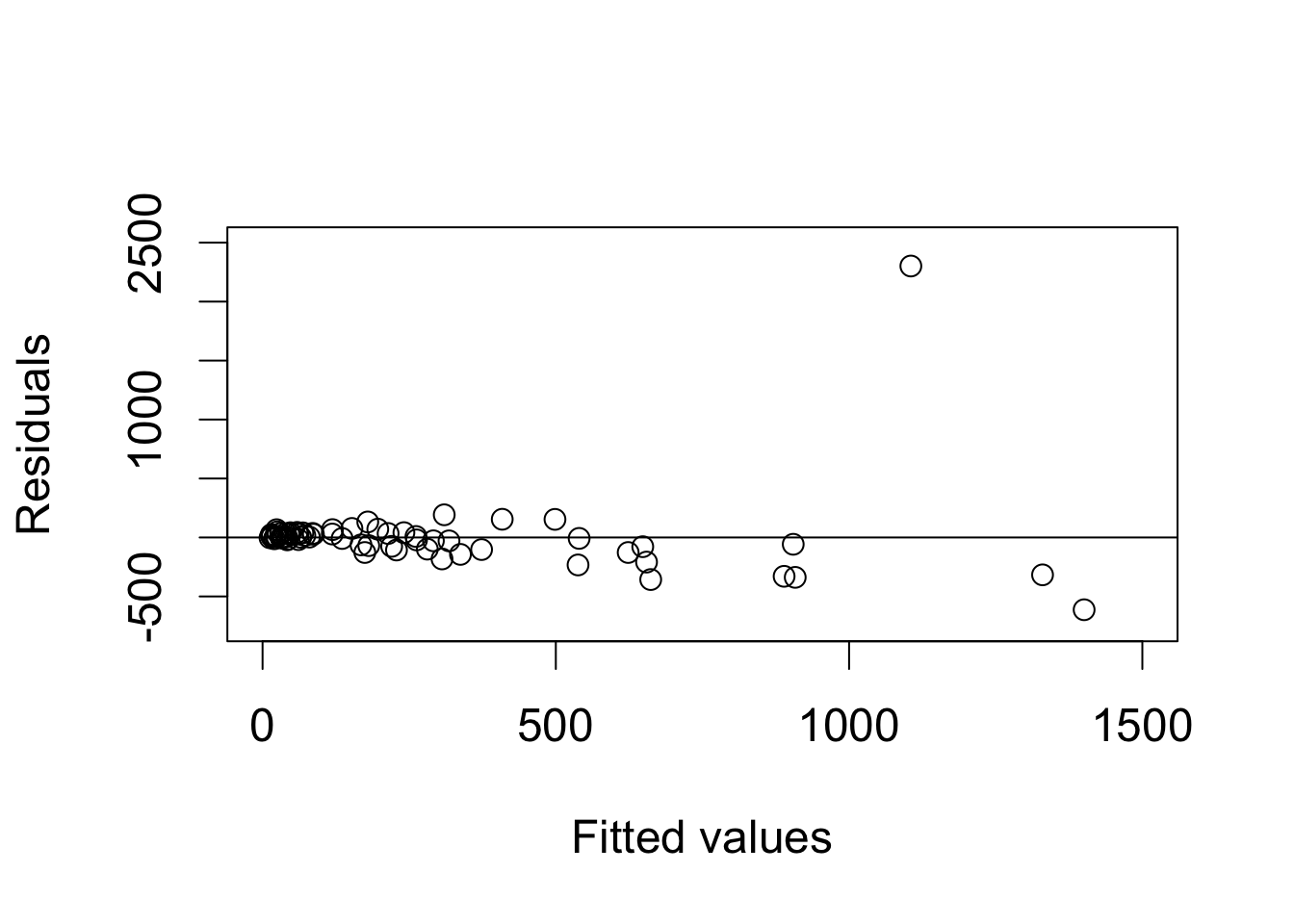florida$county[resid(fit2) == max(resid(fit2))] ##  "PalmBeach" ## data without Palm Beach florida.pb <- subset(florida, subset = (county != "PalmBeach")) fit3 <- lm(Buchanan00 ~ Perot96, data = florida.pb) fit3 ## ## Call: ## lm(formula = Buchanan00 ~ Perot96, data = florida.pb) ## ## Coefficients: ## (Intercept) Perot96 ## 45.84193 0.02435 ## R^2 or coefficient of determination R2(fit3) ##  0.8511675 par(cex = 1.5) ## residual plot plot(fitted(fit3), resid(fit3), xlim = c(0, 1500), ylim = c(-750, 2500), xlab = "Fitted values", ylab = "Residuals", main = "Residual plot without Palm Beach") abline(h = 0) # horizontal line at 0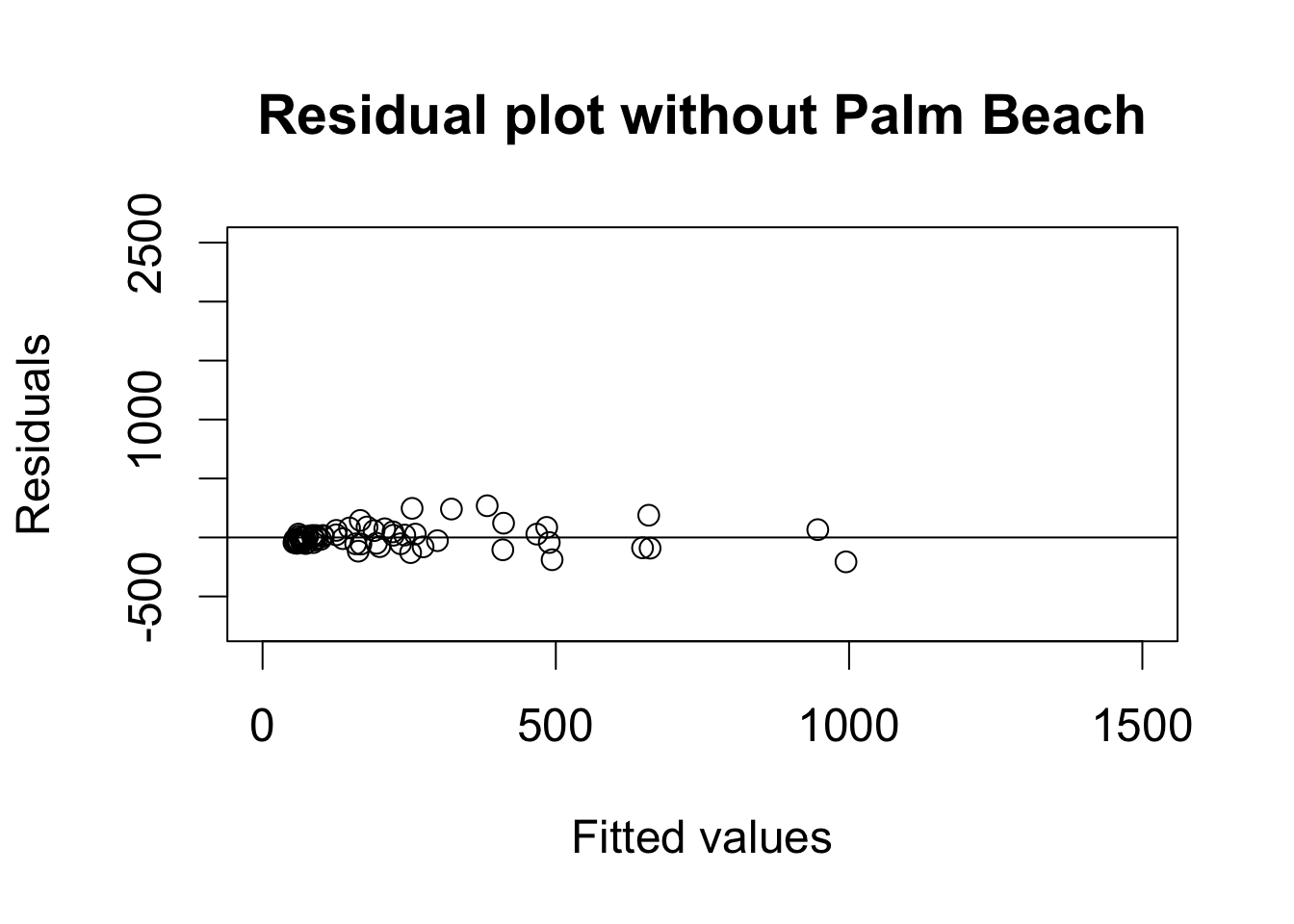plot(florida$Perot96, florida$Buchanan00, xlab = "Perot's votes in 1996", ylab = "Buchanan's votes in 2000") abline(fit2, lty = "dashed") # regression with Palm Beach abline(fit3) # regression without Palm Beach text(30000, 3250, "Palm Beach") text(30000, 1500, "regression\n with Palm Beach") text(30000, 400, "regression\n without Palm Beach")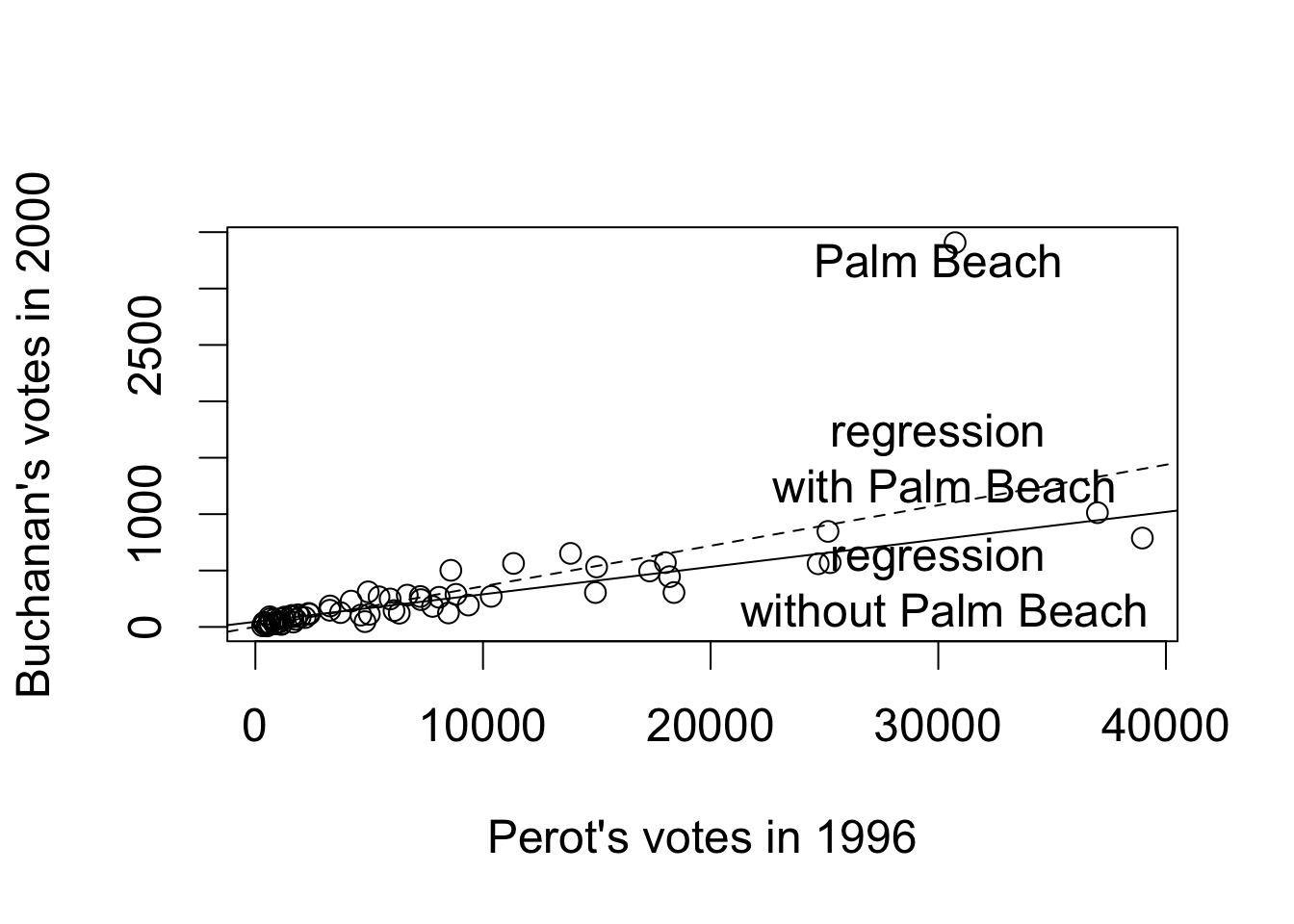## Section 4.3: Regression and Causation ### Section 4.3.1: Randomized Experiments data("women", package = "qss") ## proportion of female politicians in reserved GP vs. unreserved GP mean(women$female[women$reserved == 1]) ##  1 mean(women$female[women$reserved == 0]) ##  0.07476636 ## drinking-water facilities mean(women$water[women$reserved == 1]) - mean(women$water[women$reserved == 0]) ##  9.252423 ## irrigation facilities mean(women$irrigation[women$reserved == 1]) - mean(women$irrigation[women$reserved == 0]) ##  -0.3693319 lm(water ~ reserved, data = women) ## ## Call: ## lm(formula = water ~ reserved, data = women) ## ## Coefficients: ## (Intercept) reserved ## 14.738 9.252 lm(irrigation ~ reserved, data = women) ## ## Call: ## lm(formula = irrigation ~ reserved, data = women) ## ## Coefficients: ## (Intercept) reserved ## 3.3879 -0.3693 ### Section 4.3.2: Regression with Multiple Predictors data("social", package = "qss") levels(social$messages) # base level is Civic'
## NULL
fit <- lm(primary2006 ~ messages, data = social)
fit
##
## Call:
## lm(formula = primary2006 ~ messages, data = social)
##
## Coefficients:
##       (Intercept)    messagesControl  messagesHawthorne
##          0.314538          -0.017899           0.007837
## messagesNeighbors
##          0.063411
## ## create indicator variables
## social$Control <- ifelse(social$messages == "Control", 1, 0)
## social$Hawthorne <- ifelse(social$messages == "Hawthorne", 1, 0)
## social$Neighbors <- ifelse(social$messages == "Neighbors", 1, 0)
## ## fit the same regression as above by directly using indicator variables
## lm(primary2006 ~ Control + Hawthorne + Neighbors, data = social)

## create a data frame with unique values of messages'
unique.messages <- data.frame(messages = unique(social$messages)) unique.messages ## messages ## 1 Civic Duty ## 2 Hawthorne ## 3 Control ## 4 Neighbors ## make prediction for each observation from this new data frame predict(fit, newdata = unique.messages) ## 1 2 3 4 ## 0.3145377 0.3223746 0.2966383 0.3779482 ## sample average tapply(social$primary2006, social$messages, mean) ## Civic Duty Control Hawthorne Neighbors ## 0.3145377 0.2966383 0.3223746 0.3779482 ## linear regression without intercept fit.noint <- lm(primary2006 ~ -1 + messages, data = social) fit.noint ## ## Call: ## lm(formula = primary2006 ~ -1 + messages, data = social) ## ## Coefficients: ## messagesCivic Duty messagesControl messagesHawthorne ## 0.3145 0.2966 0.3224 ## messagesNeighbors ## 0.3779 ## estimated average effect of Neighbors' condition coef(fit)["messagesNeighbors"] - coef(fit)["messagesControl"] ## messagesNeighbors ## 0.08130991 ## difference in means mean(social$primary2006[social$messages == "Neighbors"]) - mean(social$primary2006[social$messages == "Control"]) ##  0.08130991 ## adjusted Rsquare adjR2 <- function(fit) { resid <- resid(fit) # residuals y <- fitted(fit) + resid # outcome n <- length(y) TSS.adj <- sum((y - mean(y))^2) / (n - 1) SSR.adj <- sum(resid^2) / (n - length(coef(fit))) R2.adj <- 1 - SSR.adj / TSS.adj return(R2.adj) } adjR2(fit) ##  0.003272788 R2(fit) # unadjusted Rsquare calculation ##  0.003282564 summary(fit)$adj.r.squared
##  0.003272788

### Section 4.3.3: Heterogenous Treatment Effects

## average treatment effect (ate) among those who voted in 2004 primary
social.voter <- subset(social, primary2004 == 1)

ate.voter <-
mean(social.voter$primary2006[social.voter$messages == "Neighbors"]) -
mean(social.voter$primary2006[social.voter$messages == "Control"])
ate.voter
##  0.09652525
## average effect among those who did not vote
social.nonvoter <- subset(social, primary2004 == 0)

ate.nonvoter <-
mean(social.nonvoter$primary2006[social.nonvoter$messages == "Neighbors"]) -
mean(social.nonvoter$primary2006[social.nonvoter$messages == "Control"])
ate.nonvoter
##  0.06929617
## difference
ate.voter - ate.nonvoter
##  0.02722908
## subset neighbors and control groups
social.neighbor <- subset(social, (messages == "Control") |
(messages == "Neighbors"))

## standard way to generate main and interaction effects
fit.int <- lm(primary2006 ~ primary2004 + messages + primary2004:messages,
data = social.neighbor)
fit.int
##
## Call:
## lm(formula = primary2006 ~ primary2004 + messages + primary2004:messages,
##     data = social.neighbor)
##
## Coefficients:
##                   (Intercept)                    primary2004
##                       0.23711                        0.14870
##             messagesNeighbors  primary2004:messagesNeighbors
##                       0.06930                        0.02723
## lm(primary2006 ~ primary2004 * messages, data = social.neighbor)

social.neighbor$age <- 2008 - social.neighbor$yearofbirth
summary(social.neighbor$age) ## Min. 1st Qu. Median Mean 3rd Qu. Max. ## 22.00 43.00 52.00 51.82 61.00 108.00 fit.age <- lm(primary2006 ~ age * messages, data = social.neighbor) fit.age ## ## Call: ## lm(formula = primary2006 ~ age * messages, data = social.neighbor) ## ## Coefficients: ## (Intercept) age messagesNeighbors ## 0.0894768 0.0039982 0.0485728 ## age:messagesNeighbors ## 0.0006283 ## age = 25, 45, 65, 85 in Neighbors group age.neighbor <- data.frame(age = seq(from = 25, to = 85, by = 20), messages = "Neighbors") ## age = 25, 45, 65, 85 in Control group age.control <- data.frame(age = seq(from = 25, to = 85, by = 20), messages = "Control") ## average treatment effect for age = 25, 45, 65, 85 ate.age <- predict(fit.age, newdata = age.neighbor) - predict(fit.age, newdata = age.control) ate.age ## 1 2 3 4 ## 0.06428051 0.07684667 0.08941283 0.10197899 fit.age2 <- lm(primary2006 ~ age + I(age^2) + messages + age:messages + I(age^2):messages, data = social.neighbor) fit.age2 ## ## Call: ## lm(formula = primary2006 ~ age + I(age^2) + messages + age:messages + ## I(age^2):messages, data = social.neighbor) ## ## Coefficients: ## (Intercept) age ## -9.700e-02 1.172e-02 ## I(age^2) messagesNeighbors ## -7.389e-05 -5.275e-02 ## age:messagesNeighbors I(age^2):messagesNeighbors ## 4.804e-03 -3.961e-05 ## predicted turnout rate under the Neighbors'' treatment condition yT.hat <- predict(fit.age2, newdata = data.frame(age = 25:85, messages = "Neighbors")) ## predicted turnout rate under the control condition yC.hat <- predict(fit.age2, newdata = data.frame(age = 25:85, messages = "Control")) par(cex = 1.5) ## plotting the predicted turnout rate under each condition plot(x = 25:85, y = yT.hat, type = "l", xlim = c(20, 90), ylim = c(0, 0.5), xlab = "Age", ylab = "Predicted turnout rate") lines(x = 25:85, y = yC.hat, lty = "dashed") text(40, 0.45, "Neighbors condition") text(45, 0.15, "Control condition")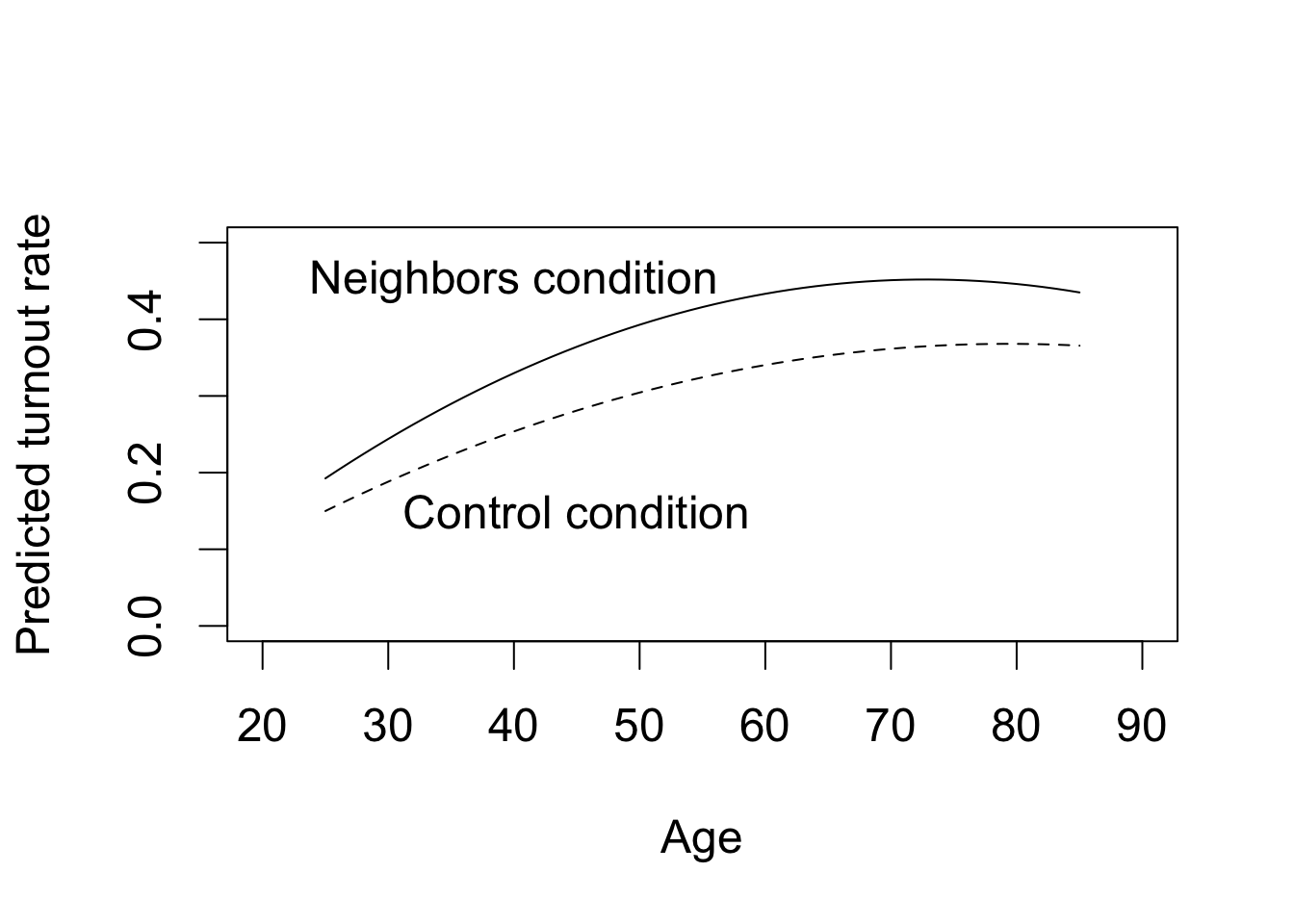## plotting the average treatment effect as a function of age plot(x = 25:85, y = yT.hat - yC.hat, type = "l", xlim = c(20, 90), ylim = c(0, 0.1), xlab = "Age", ylab = "Estimated average treatment effect")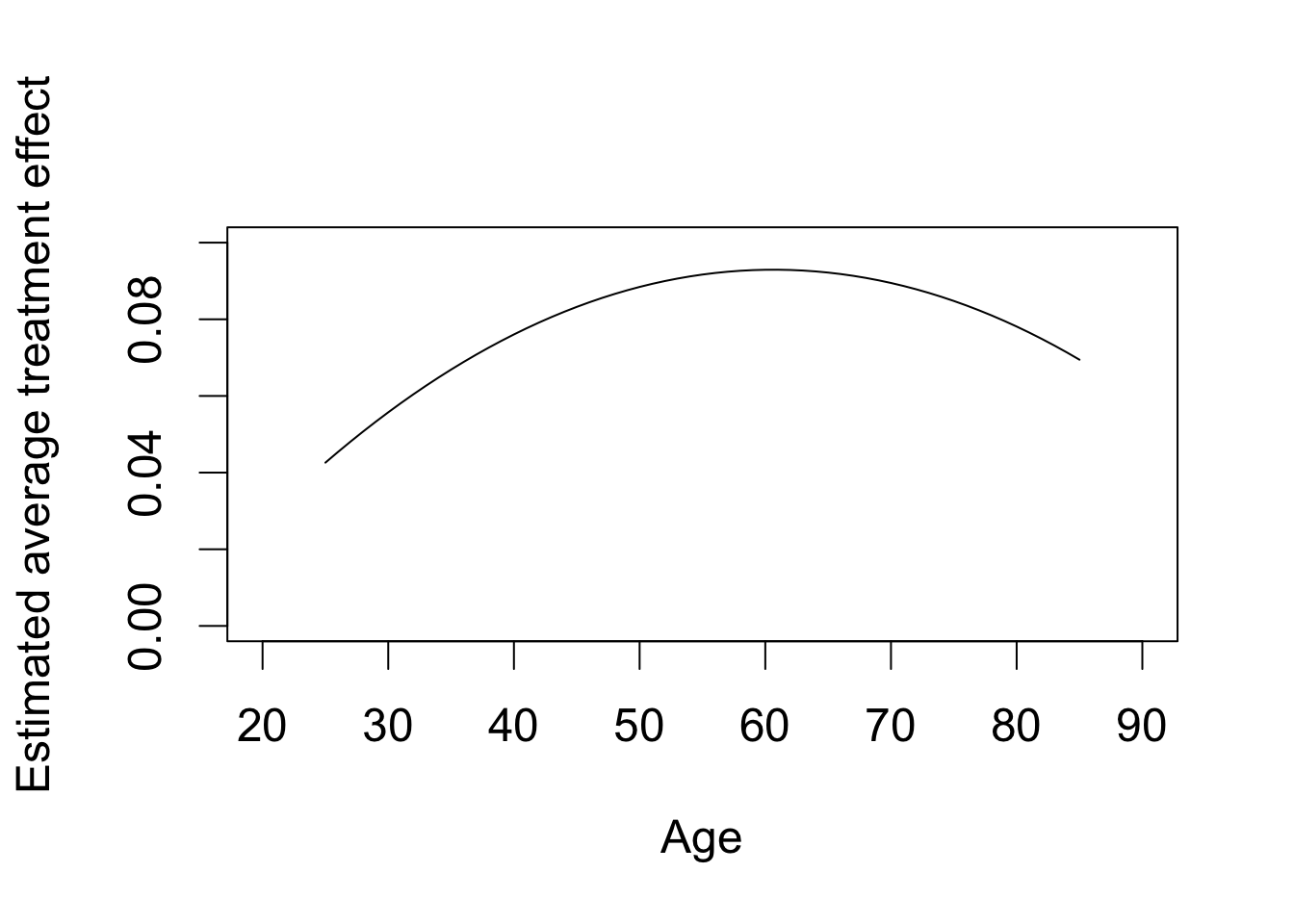### Section 4.3.4: Regression Discontinuity Design ## load the data and subset them into two parties data("MPs", package = "qss") MPs.labour <- subset(MPs, subset = (party == "labour")) MPs.tory <- subset(MPs, subset = (party == "tory")) ## two regressions for Labour: negative and positive margin labour.fit1 <- lm(ln.net ~ margin, data = MPs.labour[MPs.labour$margin < 0, ])
labour.fit2 <- lm(ln.net ~ margin,
data = MPs.labour[MPs.labour$margin > 0, ]) ## two regressions for Tory: negative and positive margin tory.fit1 <- lm(ln.net ~ margin, data = MPs.tory[MPs.tory$margin < 0, ])
tory.fit2 <- lm(ln.net ~ margin, data = MPs.tory[MPs.tory$margin > 0, ]) ## Labour: range of predictions y1l.range <- c(min(MPs.labour$margin), 0) # min to 0
y2l.range <- c(0, max(MPs.labour$margin)) # 0 to max ## prediction y1.labour <- predict(labour.fit1, newdata = data.frame(margin = y1l.range)) y2.labour <- predict(labour.fit2, newdata = data.frame(margin = y2l.range)) ## Tory: range of predictions y1t.range <- c(min(MPs.tory$margin), 0) # min to 0
y2t.range <- c(0, max(MPs.tory$margin)) # 0 to max ## predict outcome y1.tory <- predict(tory.fit1, newdata = data.frame(margin = y1t.range)) y2.tory <- predict(tory.fit2, newdata = data.frame(margin = y2t.range)) par(cex = 1.5) ## scatterplot with regression lines for labour plot(MPs.labour$margin, MPs.labour$ln.net, main = "Labour", xlim = c(-0.5, 0.5), ylim = c(6, 18), xlab = "Margin of victory", ylab = "log net wealth at death") abline(v = 0, lty = "dashed") ## add regression lines lines(y1l.range, y1.labour, col = "red") lines(y2l.range, y2.labour, col = "red")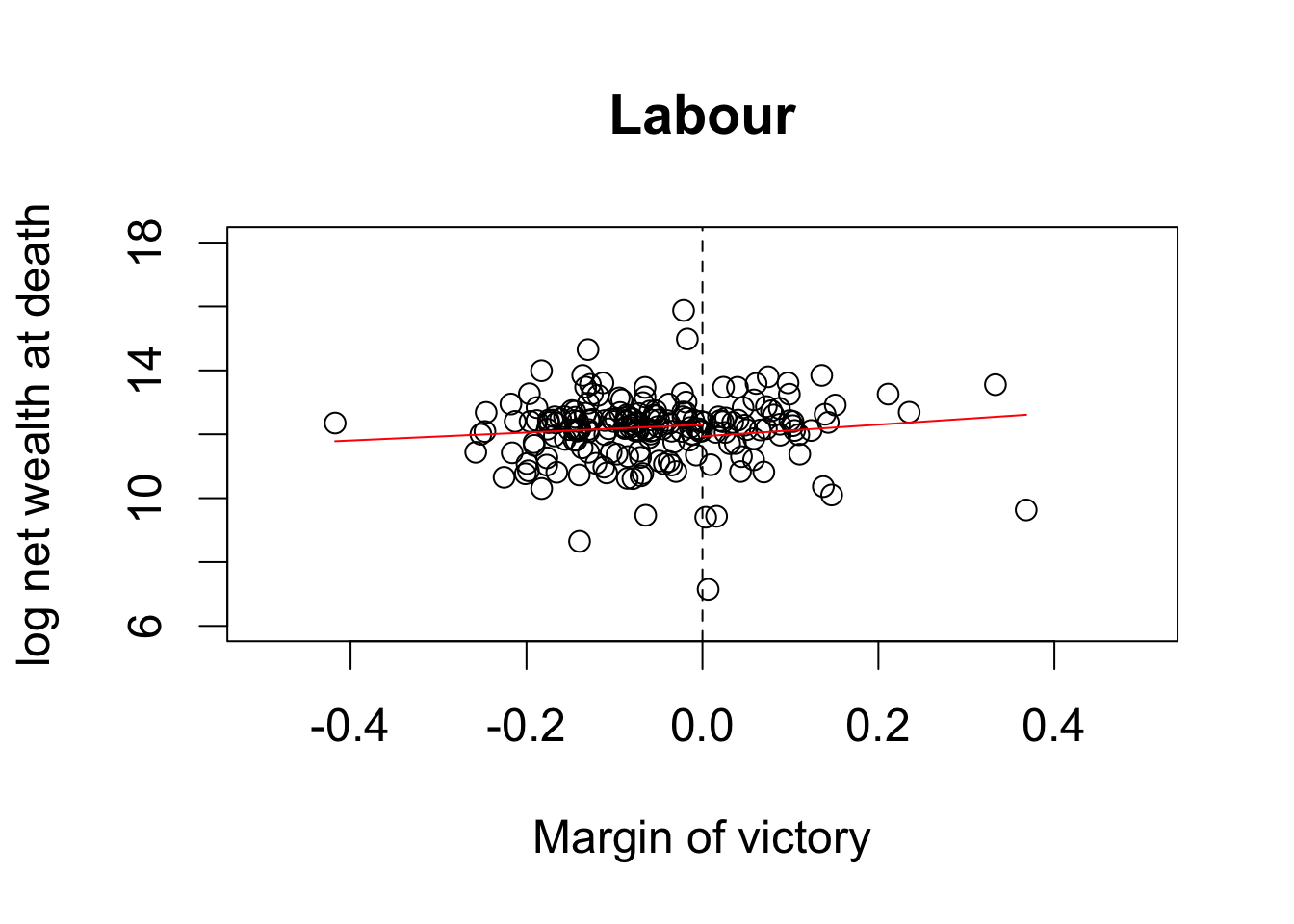## scatterplot with regression lines for tory plot(MPs.tory$margin, MPs.tory$ln.net, main = "Tory", xlim = c(-0.5, 0.5), ylim = c(6, 18), xlab = "Margin of victory", ylab = "log net wealth at death") abline(v = 0, lty = "dashed") ## add regression lines lines(y1t.range, y1.tory, col = "red") lines(y2t.range, y2.tory, col = "red")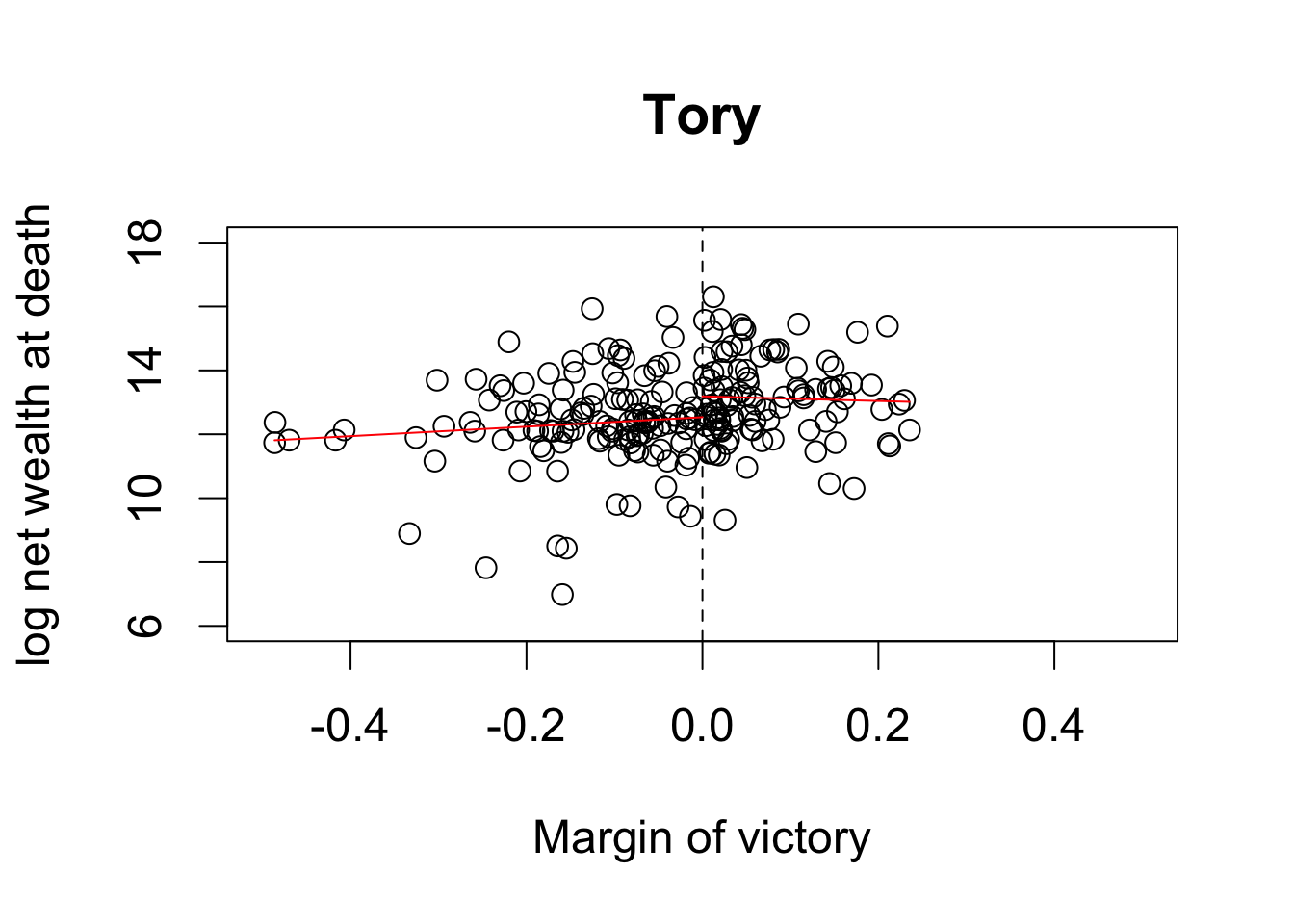## average net wealth for Tory MP tory.MP <- exp(y2.tory) tory.MP ## 1 ## 533813.5 ## average net wealth for Tory non-MP tory.nonMP <- exp(y1.tory) tory.nonMP ## 2 ## 278762.5 ## causal effect in pounds tory.MP - tory.nonMP ## 1 ## 255050.9 ## two regressions for Tory: negative and positive margin tory.fit3 <- lm(margin.pre ~ margin, data = MPs.tory[MPs.tory$margin < 0, ])
tory.fit4 <- lm(margin.pre ~ margin, data = MPs.tory[MPs.tory\$margin > 0, ])
## the difference between two intercepts is the estimated effect
coef(tory.fit4) - coef(tory.fit3)
## (Intercept)
## -0.01725578`